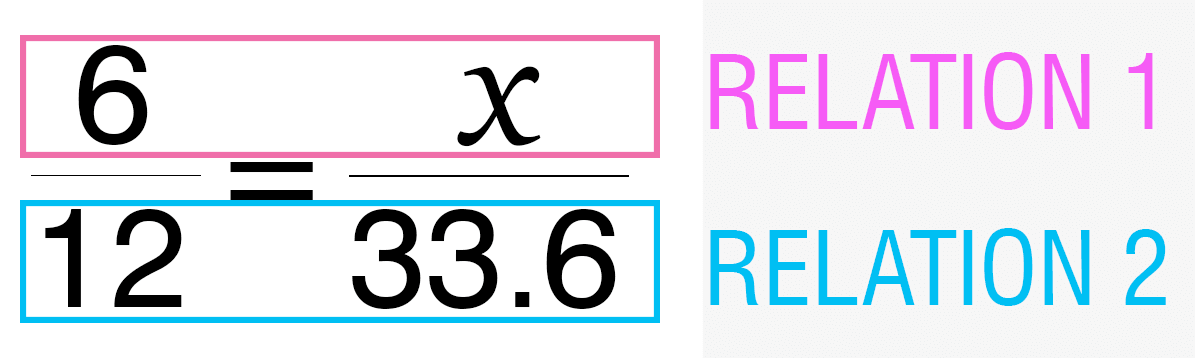BIG IDEAS:(taken from “Big Ideas by Dr. Small”):

1. Proportional thinking involves the use of multiplicative relationships, in the form of rates, ratios, and percents, to solve problems.
2. A ratio is a comparison between two numbers. A part-to-part ratio compares two parts of something. A part-to-whole ratio compares one part of something to the whole thing.
3. A rate is a ratio that involves two quantities with different units. In order to compare rates, you must make the second value the same (e.g. unit rates).
4. A percent is a ratio where the second number is 100.

STUDENT LEARNING GOALS:

GOAL #1: I can represent and identify ratios.

GOAL #2: I can solve problems involving proportions.

GOAL #3: I can solve problems involving rates.

GOAL #4: I can solve problems involving percents.

CURRICULUM EXPECTATIONS:

• Translate between equivalent forms of a number (i.e., decimals, fractions, percents)
• Solve problems involving percents expressed to one decimal place (e.g., 12.5%) and whole-number percents greater than 100 (e.g., 115%) (Sample problem:The total cost of an item with tax included [115%] is \$23.00. Use base ten materials to determine the price before tax.);
• Identify and describe real-life situations involving two quantities that are directly proportional (e.g., the number of servings and the quantities in a recipe, mass and volume of a substance, circumference and diameter of a circle);
• Solve problems involving proportions, using concrete materials, drawings, and variables (Sample problem:The ratio of stone to sand in HardFast Concrete is 2 to 3. How much stone is needed if 15 bags of sand are used?);
• Solve problems involving percent that arise from real-life contexts (e.g., discount, sales tax, simple interest) (Sample problem: In Ontario, people often pay a provincial sales tax [PST] of 8% and a federal sales tax [GST] of 7% when they make a purchase. Does it matter which tax is calculated first? Explain your reasoning.);
• Solve problems involving rates (Sample problem: A pack of 24 CDs costs \$7.99. A pack of 50 CDs costs \$10.45. What is the most economical way to purchase 130 CDs?).